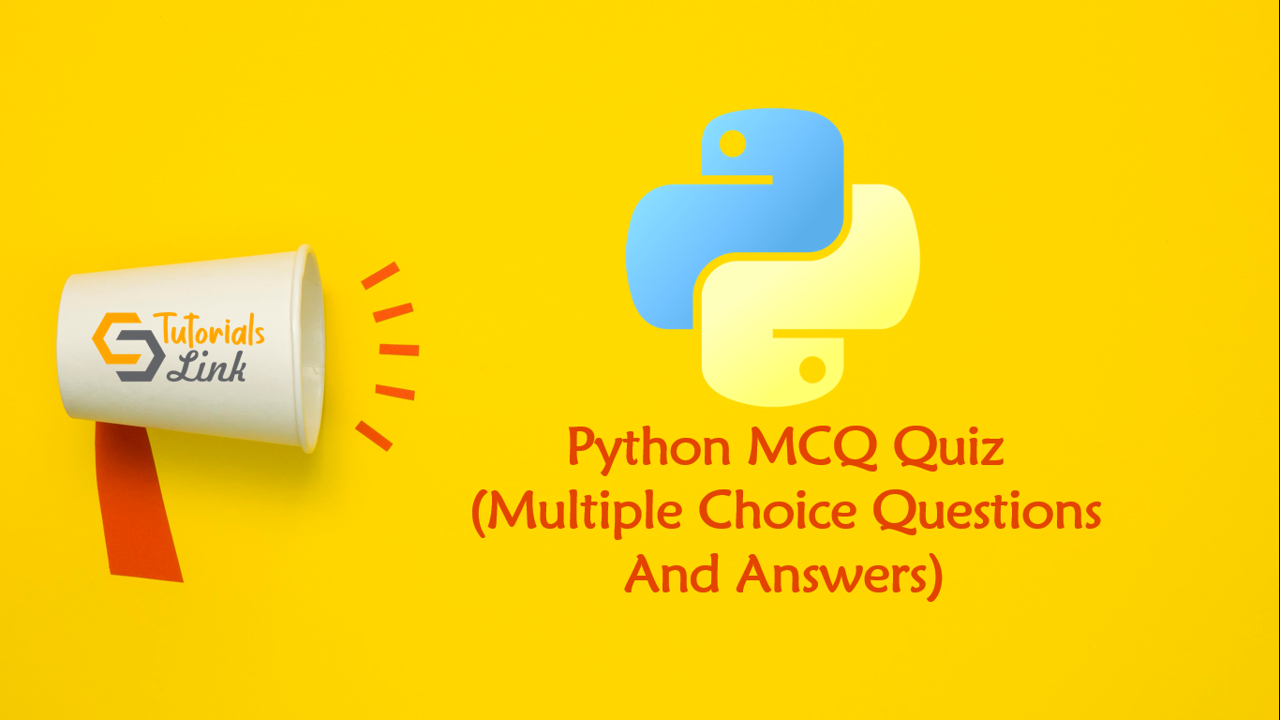Loading, please wait...# Python Multiple Choice Questions And Answers

Search here for MCQs

Are you preparing for the next job interviews? If yes, trust me this post will help you also we'll suggest you check out a big collection for Programming Full Forms that may help you in your interview:

## 6. What will be the output of the following Python code?

``````example = "snow world"

print("%s" % example[4:7])``````

Execute in the shell and verify.

• wo
• world
• sn
• rl

## 7. What will be the output of the following Python code?

``max("what are you")``

Max returns the character with the highest ascii value.

• error
• u
• t
• y

## 8. Given a string example=”hello” what is the output of example.count(‘l’)?

l occurs twice in hello.
• 2
• 1
• None
• 0

## 9. What will be the output of the following Python code?

``````example = "helle"
example.rfind("e")``````

Returns highest index.

• -1
• 4
• 1

## 10. Read the information given below carefully and write a list comprehension such that the output is: [‘e’, ‘o’]

``````w="hello"
v=('a', 'e', 'i', 'o', 'u')``````

The tuple ‘v’ is used to generate a list containing only vowels in the string ‘w’. The result is a list containing only vowels present in the string “hello”. Hence the required list comprehension is: [x for x in w if x in v].

• [x for w in v if x in v]
• [x for x in w if x in v]
• [x for x in v if w in v]
• [x for v in w for x in w]